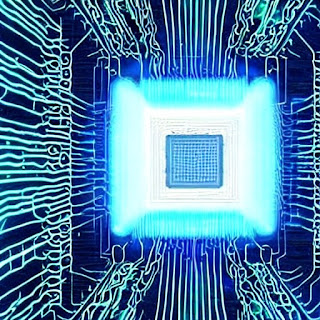# Quantum Computing BasicsQuantum Computing Basics

Question: What is a quantum computer?
Answer: A quantum computer is a computer that uses the principles of quantum mechanics to solve problems that are too complex for classical computers.
Question: What are qubits?
Answer: Qubits are the basic units of information in a quantum computer. They can be in a superposition of states, meaning that they can be both 0 and 1 at the same time.
Question: What are some potential applications of quantum computing?
Answer: Some potential applications of quantum computing include breaking encryption algorithms, simulating complex chemical reactions, designing new drugs, forecasting the weather, and developing new materials.
Question: What are some of the challenges that need to be overcome before quantum computers can be widely used?
Answer: Some of the challenges that need to be overcome before quantum computers can be widely used include noise, scalability, and decoherence.
Question: What are some of the leading companies working on quantum computing?
Answer: Some of the leading companies working on quantum computing include Google, IBM, Microsoft, and Rigetti Computing.
Question: What are some of the academic institutions working on quantum computing?
Answer: Some of the academic institutions working on quantum computing include the University of California, Berkeley, the Massachusetts Institute of Technology, and Stanford University.
Question: What is the future of quantum computing?
Answer: The future of quantum computing is very promising. There is a lot of potential for quantum computers to revolutionize many industries, and the field is rapidly advancing.
Question: What is the difference between quantum computing and classical computing?
Answer: Quantum computing and classical computing are two fundamentally different ways of computing. Classical computers use bits, which can be either 0 or 1. Quantum computers use qubits, which can be in a superposition of states, meaning that they can be both 0 and 1 at the same time.
Question: How does quantum computing work?
Answer: Quantum computing works by using the principles of quantum mechanics to manipulate qubits. Qubits can be entangled, which means that they are linked together in such a way that they share the same fate. This allows quantum computers to perform certain calculations exponentially faster than classical computers.
Question: What are the challenges of quantum computing?
Answer: There are a number of challenges that need to be overcome before quantum computing can be widely used. These challenges include noise, scalability, and decoherence.
Question: What is noise?
Answer: Noise is a random disturbance that can interfere with the operation of a quantum computer. Noise can be caused by a number of factors, including environmental factors, such as heat and vibration, and the interaction of qubits with each other.
Question: What is scalability?
Answer: Scalability is the ability to increase the number of qubits in a quantum computer without sacrificing performance. Scalability is a major challenge for quantum computing, as the number of qubits needed to solve certain problems grows exponentially.
Question: What is decoherence?
Answer: Decoherence is the loss of quantum coherence, which is the ability of qubits to be in a superposition of states. Decoherence can be caused by a number of factors, including noise and the interaction of qubits with their environment.
Question: What is the current state of quantum computing?
Answer: The current state of quantum computing is still in its early stages. However, there has been a lot of progress in recent years, and there is a lot of optimism that quantum computers will be developed in the near future.